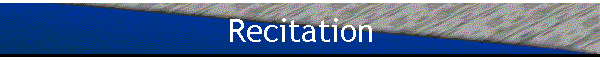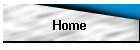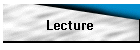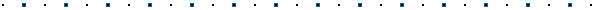Analytical program of recitations - Weekly schedule1st week Topics: Methodology Basic points: Examples: Applications 2nd week Topics: Methodology Basic points: Examples: Applications 3rd week Topics: Methodology Basic points: Examples: Applications 4th week Topics: Methodology Basic points: Examples: Applications 5th week Topics: Methodology Basic points: Examples: Applications 6th week Topics: Methodology Basic points: Examples: Applications: 7th week Topics: Methodology Basic points: Examples: Applications 8th week Topics: Methodology Basic points: Examples: Applications: 9th week Topics: Methodology Basic points: Examples: Applications 10th week Topics: Methodology Basic points: Examples: Applications 11th week Topics: Methodology Basic points: Examples: Applications 12th week Topics: Methodology Basic points: Examples: Applications 13th week Topics: Methodology Basic points: Examples: Applications 14thweek Topics: Methodology Basic points: Examples: Applications 15th week Final Examination